# Samacheer Kalvi Books: Tamilnadu State Board Text Books Solutions

## Samacheer Kalvi 12th Economics Chapter 4 Consumption and Investment Functions Notes PDF Download: Tamil Nadu STD 12th Economics Chapter 4 Consumption and Investment Functions NotesSamacheer Kalvi 12th Economics Chapter 4 Consumption and Investment Functions Notes PDF Download: Tamil Nadu STD 12th Economics Chapter 4 Consumption and Investment Functions Notes

Samacheer Kalvi 12th Economics Chapter 4 Consumption and Investment Functions Notes PDF Download: Students of class can download the Samacheer Kalvi 12th Economics Chapter 4 Consumption and Investment Functions Notes PDF Download from our website. We have uploaded the Samacheer Kalvi 12th Economics Chapter 4 Consumption and Investment Functions notes according to the latest chapters present in the syllabus. Download Samacheer Kalvi 12th Economics Chapter 4 Consumption and Investment Functions Chapter Wise Notes PDF from the links provided in this article.

## Samacheer Kalvi 12th Economics Chapter 4 Consumption and Investment Functions Notes PDF Download

We bring to you specially curated Samacheer Kalvi 12th Economics Chapter 4 Consumption and Investment Functions Notes PDF which have been prepared by our subject experts after carefully following the trend of the exam in the last few years. The notes will not only serve for revision purposes, but also will have several cuts and easy methods to go about a difficult problem.

 Board Tamilnadu Board Study Material Notes Class Samacheer Kalvi 12th Economics Subject 12th Economics Chapter Chapter 4 Consumption and Investment Functions Format PDF Provider Samacheer Kalvi Books

## How to Download Samacheer Kalvi 12th Economics Chapter 4 Consumption and Investment Functions Notes PDFs?

2. Click on the Samacheer Kalvi 12th Economics Notes PDF.
3. Look for your preferred subject.
4. Now download the Samacheer Kalvi 12th Economics Chapter 4 Consumption and Investment Functions notes PDF.

## Download Samacheer Kalvi 12th Economics Chapter 4 Consumption and Investment Functions Chapterwise Notes PDF

PART – A

Multiple Choice questions

Question 1.
The average propensity to consume is measured by
a) C\Y
b) C x Y
c) Y\C
d) C + Y
a) C\Y

Question 2.
An increase in the marginal propensity to consume will:
a) Lead to consumption function becoming steeper
b) Shift the consumption function upwards
c) Shift the consumption function downwards
d) Shift savings function upwards
a) Lead to consumption function becoming steeper

Question 3.
If the Keynesian consumption function is C = 10 + 0.8 Y then, if the disposable income is Rs 1000, What is the amount of total consumption?
a) ₹ 0.8
b) ₹ 800
c) ₹ 810
d) ₹ 081
c) ₹ 810

Question 4.
If the Keynesian consumption function is C = 10 + 0.8 Y then when disposable income is Rs 100, What is the marginal propensity to consume?
a) ₹ 0.8
b) ₹ 800
c) ₹810
d) ₹0.81
a) ₹ 0.8

Question 5.
If the Keynesian consumption function is C = 10 + 0.8 Y then, and disposable income is ₹ 100, what is the average propensity to consume?
a) ₹ 0.8
b) ₹ 800
c) ₹ 810
d) ₹ 50.9
d) ₹ 50.9

Question 6.
As national income increases
a) The APC fall’s and gets nearer in value to the MPC
b) The APC increases and diverges in value from the MPC
c) The APC stays constant
d) The APC always approaches infinity.
a) The APC fall’s and gets nearer in value to the MPC

Question 7.
An increase in consumption at any given level of income is likely to lead
a) Higher aggregate demand
b) An increase in exports
c) A fall in taxation revenue
d) A decrease in import spending
a) Higher aggregate demand

Question 8.
Lower interest rates are likely to :
a) Decrease in consumption
b) increase the cost of borrowing
c) Encourage saving
d) increase borrowing and spending
d) increase borrowing and spending

Question 9.
The MPC is equal to :
a) Total spending / total consumption
b) Total consumption / total income
c) Change in consumption/ change in income.
d) none of the above.
c) Change in consumption/ change in income.

Question 10.
The relationship between total spending on consumption and the total income is the ………………………
a) Consumption function
b) Savings function
c) Investment function
d) aggregate demand function
a) Consumption function

Question 11.
The sum of the MPC and MPS is ……………..
a) 1
b) 2
c) 0.1
d) 1.1
a) 1

Question 12.
As income increases, consumption will ……………..
a) Fall
b) not change
c) fluctuate
d) increase
d) increase

Question 13.
When the investment is assumed autonomous the slope of the AD schedule is determined by the …………………
a) marginal propensity to invest
b) disposable income
c) marginal propensity to consume
d) average propensity to consume.
c) marginal propensity to consume

Question 14.
The multiplier tells us how many…………………… changes after a shift in
a) Consumption, income
b) investment, output
c) savings, investment
d) output, aggregate demand
d) output, aggregate demand

Question 15.
The multiplier is calculated as
a) 1(1-MPC)
b) 1/ MPS
c) 1/ MPC
d) a and b
d)a and b

Question 16.
If the MPC is 0.5, the multiplier is …………………
a) 2
b) 1/2
c) 0.2
d) 20
a) 2

Question 17.
In an open economy import …………….. the value of the multiplier.
a) Reduces
b) increase
c) does not change
d) Changes
a) Reduces

Question 18.
According to Keynes, investment is a function of the MEC and …………………
a) Demand
b) Supply
c) Income
d) Rate of interest
d) Rate of interest

Question 19.
The term Super multiplier was first used by
a) J.R. Hicks
b) R.G.D Allen
c) Kahn
d) J.M. Keynes
a) J.R. Hicks

Question 20.
The term MEC was introduced by.
b) J.M. Keynes
c) Ricardo
d) Malthus
b) J.M. Keynes

PART – B (Two Mark Questions)

Answer the following questions in one or two sentences.

Question 21.
What is consumption function?
The consumption function is a functional relationship between total consumption and gross national income.

Question 22.
What do you mean by a propensity to Consume?
The propensity to consume refers to the income consumption relationship.

Question 23.
Define average propensity to consume (APC)
Average Propensity to Consume: The average propensity to consume is the ratio of consumption expenditure to any particular level of income.” Algebraically it may be expressed as under:
Where, C = Consumption; Y = Income
APC = CY
Where, C = Consumption; Y = Income.

Question 24.
Define marginal propensity to consume (MPC)
MPC may be defined as the ratio of the change in consumption to the change in income.
(°r)
MPC =ΔC / ΔY
ΔC = Change in consumption
ΔY = change in income

Question 25.
What do you mean by a propensity to save?

1. Thus the consumption function measures not only the amount spent on consumption but also the amount saved.
2. This is because the propensity to save is merely the propensity not to consume.
3. The 45° line may therefore be regarded as a zero – saving line, and the shape and position of the C curve indicate the division of income between consumption and saving.

Question 26.
Define average propensity to save (APS)
The average propensity to save is the ratio of saving to income.

Question 27.
Define marginal propensity to save (MPS).
Marginal Propensity to Save (MPS): Marginal Propensity to Save is the ratio of change in saving to a change in income.
MPS is obtained by dividing change in savings by change in income. It can be expressed algebraically as MPS = ΔSΔY
∆S = Change in Saving; ∆Y = Change in Income
Since MPC + MPS = 1
MPS = 1 – MPC and MPC = 1 – MPS.

Question 28.
Define Multiplier.
The multiplier is defined as the ratio of the change in national income to change in investment.
K=ΔYΔI

Question 29.
Define Accelerator.

1. “The accelerator coefficient is the ratio between induced investment and an initial change in consumption.”
2. Assuming the expenditure of ₹50 crores on consumption goods, if industries lead to an investment of ₹100 crores in investment goods industries, we can say that the accelerator is 2.
3. Accelerator = 100ΔY = 2

PART – C

Answer the following questions in one paragraph.

Question 30.
State the propositions of Keynes’s psychological law of consumption.
This law has three propositions:

1. when income increases, consumption expenditure also increases but by a smaller amount.
2. The increased income will be divided in some proportion between consumption expenditure and saving.
3. Increases in income always lead to an increase in both consumption and saving.

Question 31.
Differentiate autonomous and induced investment.

 Autonomous Investment Induced Investment 1. Independent Planned 2. Íncome inelastic Income elastic 3. Welfare motive Profit motive

Autonomous Investment

Induced Investment

• Independent Planned
• Íncome inelastic Income elastic
• Welfare motive Profit motive

Question 32.
Explain any three subjective and objective factors influencing the consumption function.
Subjective Factors:

1. The motive of precaution: To build up a reserve against unforeseen contingencies. e.g. Accidents, sickness. ,
2. The motive of foresight: The desire to provide for anticipated future needs. e.g. Old age.
3. The motive of calculation: The desire to enjoy interest and appreciation. Consumption and Investment Functions.

Objective Factors:
1. Income Distribution:
If there is large disparity between rich and poor, the consumption is low because the rich people have low propensity to consume and high propensity to save.

2. Price level:
Price level plays an important role in determining the consumption function. When the price falls, real income goes up; people will consume more and the propensity to save of society increases.

3. Wage level:
Wage level plays an important role in determining the consumption function and there is a positive relationship between wage and consumption. Consumption expenditure increases with the rise in wages. Similar is the effect with regard to windfall gains.

Question 33.
Mention the differences between accelerator and multiplier effect.

 Accelerator Multiplier 1. The ratio of the net change in investment to changes in consumption. The ratio of the change in national income to change in investment. 2. K=ΔYΔI β=ΔIΔC

Question 34.
State the concept of the super multiplier.

• The concept of a super multiplier was developed by Hicks. He has combined multiplier and accelerator mathematically and named it the super multiplier.
• The super multiplier is worked out by combining both consumption and induced investment.
• The super multiplier which includes induced investment is greater than the simple multiplier.

Question 35.
Specify the leakages of the multiplier.

• There is a change in autonomous investment.
• There is no induced investment
• The marginal propensity to consume is constant.
• Consumption is a function of current income.
• There are no time lags in the multiplier process.
• Consumer goods are available in response to effective demand for them.
• There is a closed economy unaffected by foreign influences.
• There are no changes in prices.
• There is less than a full-employment level in the economy.

PART – D

Answer the following questions on a page.

Question 36.
Explain Keynes’s psychological law of consumption function with a diagram.
Keynes’s psychological law of consumption function implies that there is a tendency on the part of the people to spend on consumption less than the full increment of income.
Assumptions:

1. Ceteris paribus
2. Existence of Normal conditions
3. Existence of a laissez-faire capitalist Economy

Propositions of the law:

1. When income increases, consumption expenditure also increases but by a smaller amount.
2. The increased income will be divided in some proportion between consumption expenditure and saving.
3. An increase in income always leads to an increase in both consumption and saving.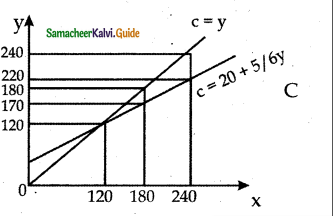The three propositions are explained in the figure. Income is measured horizontally and consumption and saving on the vertical axis. is the consumption function curve and 45° line represents income consumption equality.

Proposition (1):
When income increases from 120 to 180 consumption also increases from 120 to 170 but the increase in consumption is less than the increase in income. 10 is saved.

Proposition (2):
When income increases to 18 and 240 it is divided in some proportion between consumption by 170 and 220 and saving by 10 and 20 respectively.

Proposition (3):
Increases in income to 180 and 240 lead to increased consumption 170 and 220 and increased saving 20 and 10 than before. It is clear from the widening area below the C curve and the saving gap between 45° line and the C curve.

Question 37.
Briefly explain the subjective and objective factors of consumption function?
Subjective Factors:

1. The motive of precaution: To build up a reserve against unforeseen contingencies. e.g. Accidents, sickness
2. The motive of foresight: The desire to provide for anticipated future needs, e.g. Old age
3. The motive of calculation: The desire to enjoy interest and appreciation.
4. The motive of improvement: The desire to enjoy for improving the standard of living.
5. The motive of financial independence.
6. The motive of the enterprise (desire to do forward trading).
7. The motive of pride.(desire to bequeath a fortune)
8. The motive of avarice.(purely miserly instinct)

Objective Factors:
1. Income Distribution:
If there is large disparity between rich and poor, the consumption is low because the rich people have low propensity to consume and high propensity to save.

2. Price level:

1. Price level plays an important role in determining the consumption function.
2. When the price falls, real income goes up; people will consume more and propensity to save of the society increases.

3. Wage level:

1. Wage level plays an important role in determining the consumption function and there is positive relationship between wage and consumption.
2. Consumption expenditure increases with the rise in wages.
3. Similar is the effect with regard to windfall gains.

4. Interest rate:

1. Rate of interest plays an important role in determining the consumption function.
2. Higher rate of interest will encourage people to save more money and reduces consumption.

5. Fiscal Policy:
When the government reduces the tax the disposable income rises and the propensity to consume of community increases.

6. Consumer credit:

1. The availability of consumer credit at easy installments will encourage households to buy consumer durables like automobiles, fridges, computers.
2. This pushes up consumption.

7. Demographic factors:

1. Ceteris paribus, the larger the size of the family, the grater is the consumption.
2. Besides size of the family, stage in family life cycle, place of residence and occupation affect the consumption function.

8. Duesenberry hypothesis:
Duesenberry has made two observations regarding the factors affecting consumption.

1. The consumption expenditure depends not only on his current income but also past income and standard of living.
2. Consumption is influenced by the demonstration effect. The consumption standards of low-income groups are influenced by the consumption standards of high-income groups.

9. Windfall Gains or losses:
Unexpected changes in the stock market leading to gains or losses tend to shift the consumption function upward or downward.

Question 38.
Illustrate the working of Multiplier.
Suppose the Government undertakes investment expenditure equal to Rs.100 crore on some public works, by way of wages, price of materials etc. Thus income of labourer and suppliers increases by Rs. 100 crore.

Suppose the MPC is 0.8 that is 80% A Sum of Rs. 80.crores is spent on consump¬tion. As a result, suppliers get an income of Rs. 80 crores. They in turn spend Rs. 64 crores. In this manner consumption expenditure and increase in income act in a chain-like manner.

The final result is Δy = 100 + 100 x 45 + 100 x (45)2 + 100 x (45)3 or,
= 100 + 100 x 0.8 + 100 (0.8)2 + 100 x (0.8)3
100 +80 + 64 + 51.2
500
(ie) 100 x 1/1 – 4/5
= 100 x 1/1/5
1000 x 5 = Rs. 500 crores
For instance if C = 100 + 0.8Y, I = 100,
Then Y = 100 + 0.8y + 100
0.2y = 200
Y = 200/ 0.2 = 1000 …………….. Point B
If I is increased to 110, then
0.2 Y = 210
Y – 210 / 0.2 = 1050 ………………….Point D
For Rs 10 increase in I, Y has increased by Rs,50
This is due to multiplier effect.
At point A, Y = C= 500
C = 100 + 0.8 (500) = 500; S = 0
At point B, Y = 1000
C = 100 + 0.8 (1000) = 900 : S = 100 = I
At point D, Y = 1050
C= 100 + 0.8 (1050) = 940 : S = 110 = I

when I is increased by 10, Y increases by 50
This is multiplier effect (K = 5)
k = 10.2 = 5

Question 39.
Explain the operation of the Accelerator.
Operation of the Acceleration Principle:

1. Let us consider a simple example. The operation of the accelerator may be illustrated as follows.
2. Let us suppose that in order to produce 1000 consumer goods, 100 machines are required.
3. Also, suppose that the working life of a machine is 10 years.
4. This means that every year 10 machines have to be replaced in order to maintain the constant flow of 1000 consumer goods. This might be called replacement demand.
5. Suppose that demand for consumer goods rises by 10 percent (i.e. from 1000 to 1100).
6. This results in an increase in demand for 10 more machines.
7. So that total demand for machines is 20. (10 for replacement and 10 for meeting increased demand).
8. It may be noted here a 10 percent increase in demand for consumer goods causes a 100 percent increase in demand for machines (from 10 to 20).
9. So we can conclude even a mild change in demand for consumer goods will lead to wide change in investment.

Diagrammatic illustration:
Operation of Accelerator.

1. SS is the saving curve. II is the investment curve. At point E1 the economy is in equilibrium with OY1 income. Saving and investment are equal at OY1 Now, investment is increased from OI2 to OI4.
2. This increases income from OY1 to OY3, the equilibrium point being E3 If the increase in investment by I2 I4 is purely exogenous, then the increase in income by Y1 Y3 would have been due to the multiplier effect.
3. But in this diagram it is assumed that exogenous investment is only by I, I3 and induced investment is by I3I4.
4. Therefore, the increase in income by Y1 Y2 is due to the multiplier effect and the increase in income by Y2 Y3 is due to the accelerator effect.

Question 40.
What are the differences between MEC and MEI?

 Marginal Efficiency of capital Marginal Efficiency of Investment 1. It is based on a given supply price of capital It is based on the induced change in the price due to a change in the demand for capital 2. It represents the rate of return on all successive units of capital without regard to existing capital It shows the rate of return on just those units of capital over and above the existing capital stock 3. The capital stock is taken on the X-axis of the diagram The amount of investment is taken on the Y-axis of the diagram 4. It is a “stock” concept It is a “flow” concept 5. It determines the optimum capital stock in an economy at each level of interest rate It determines the net investment of the economy at each interest rate given the capital stock.

### 12th Economics Guide Consumption and Investment Functions Additional Important Questions and Answers

I. Match the following

Question 1.
a) Y – 1) Consumption
b) C – 2) Income
c) S – 3) Investment
d) I – 4) Savings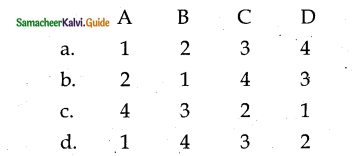b) 2 1 4 3

Question 2.
a) MPC – 1) C+S
b) K – 2) ΔI/Δ\C
c) Y – 3) ΔC/ΔY
d) 13 – 4) 1
4) 11MPC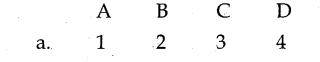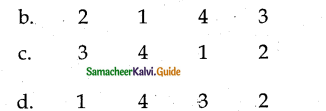c) 3 4 1 2

Question 3.
a) APC – 1) ∆S/∆Y
b) MPC – 2) S/Y
c) APS – 3) ∆C/∆Y
d) MPS – 4) C/Y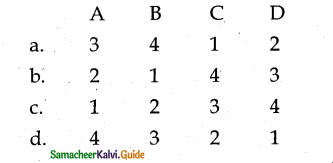d) 4 3 2 1

II. Choose the correct pair.

Question 1.
a) Multiplier – Dusenberry
b) Psychological Law of Consumption – M.F.Khan
c) Consumption function – Constant in Longrun
d) Income Multiplier – J.M.Keynes
d) Income Multiplier – J.M.Keynes

Question 2.
a) K – Accelerator
b) β – Multiplier
c) Consumption function – C= f (y)
d) Investment function – I = f (s)
c) Consumption function – C= f (y)

Question 3.
a) K – ∆Y/∆I
b) K – 11MPS
c) MEC – Flow concept
d) MEl – Stock concept
a) K – ∆Y/∆I

Question 4.
a) MPS = ∆Y/∆S
b) APS = C/Y
c) MPC = ∆C/∆Y
d) APC = S/Y
MPC = MPC=ΔCΔY

III. Choose the incorrect pair

Question 1.
a) Multiplier concept – – Mf.khan
b) Investment Multiplier – J.M.Clark
c) Income Multiplier – J.M.Keynes
d) Accelerator concept – J.B.Say
b) Investment Multiplier – J.B.Say

Question 2.
a) Y – Total Income
b) C – Savings expenditure
c) IA – Autonomous Investment
d) IP – Induced private investment
b) C – Savings expenditure

Question 3.
a) Consumption function – relation between income and consumption
b) Autonomous Investment – investment independent of change in Income
c) Super Multiplier – K and β interaction.
d) Investment function – relation between consumption and investment.
d) Investment function – relation between consumption and investment.

IV. Choose the correct statement

Question 1.
a) The primary microeconomic objective is the acceleration of the growth of national income.
b) MPC is expressed in percentage and the MPC infraction.
c) Laissez-Faire exists in a Capitalist Economy.
d) Increase in rate of interest reduces savings.
c) Laissez – Faire exists in capitalist Economy.

Question 2.
a) When the government reduces the tax the disposable income falls.
b) Autonomous investment does not depend on the national income.
c) There exists a negative relationship between the national income and induced investment.
d) Investment depended exclusively on the rate of interest.
b) Autonomous investment does not depend on the national income.

Question 3.
a) In times of economic depression, the governments try to boost the induced investment.
b) Induced investment is not profit-motivated.
c) Autonomous investment is profit-motivated.
d) Marginal Efficiency of capital refers to the annual percentage yield earned by the last additional unit of capital.
d) Marginal Efficiency of capital refers to the annual percentage yield earned by the last additional unit of capital.

V. Choose the incorrect statement

Question 1.
a) Multiplier is classified as static and Dynamic multiplier.
b) The accelerator expresses the ratio of the net change in investment to change in consumption.
c) The concept of super multiplier was introduced by J.M.Keynes.
d) The combined effect of the multiplier and the accelerator is also called the leverage effect.
c) The concept of super multiplier was introduced by J.M.Keynes.

Question 2.
a) Marginal propensity to consume is the ratio of consumption to income.
b) Marginal propensity to consume is the ratio of change in consumption to change in income.
c) Average propensity to save is the Ratio of the saving to income.
d) Marginal propensity to save is the ratio of change in saving to change in income.
a) Marginal propensity to consume is the ratio of consumption to income.

Question 3.
a) Super multiplier is the combined effect of the interaction of multiplier and accelerator.
b) Super multiplier includes both autonomous and induced investment.
c) The desire to secure liquid resources to meet emergencies is called liquidity preference.
d) The subjective factors that determine consumption function are real and measurable.
d) The subjective factors that determine consumption function are real and measurable.

VI. Pick the odd one out:

Question 1.
a) The motive of precaution
b) The motive of transaction
c) The motive of enterprise
d) The motive of avarice
b)The motive of transaction

Question 2.
a) Multiplier
b) Accelerator
c) Super multiplier
d) Consumption function
d) Consumption function

Question 3.
a) Income multiplier
b) Tax multiplier
c) Investment multiplier
d) Employment multiplier
a) Income multiplier

VII. Analyse the reason.

Question 1.
Assertion (A): The relationship between income and consumption is called as consumption function.
Reason (R): Change in Income should be equal to change in consumption.
c) (A) is true but (R)is false.

Question 2.
Assertion (A): Keynes propounded the fundamental psychological law of consumption which forms the basis of the consumption function.
Reason (R): There is a tendency on the part of the people to spend on consumption less than the full increment of income.
a) Assertion (A) and Reason (R) both are true, and (R) is the correct explanation of (A).

Options:
a) Assertion (A) and Reason (R) both are true, and (R) is the correct explanation of (A).
b) Assertion (A) and Reason (R) both are true, and (R) is not the correct explanation of (A).
c) (A) is true but (R)is false.
d) Both (A) and (R) are false.

Question 1.
Price level plays an important role in determining the ……………………
(a) Consumption function
(b) Income function
(c) Finance function
(d) Price function
(a) Consumption function

Question 2.
Objective factors are the ………………………………………… factors which are real and measurable.
a) Internal
b) External
c) Capital
d) Production
b) External

Question 3.
Keynes has divided factors influencing the consumption function into …………….
a) 4
b) 1
c) 2
d) 3
c) 2

Question 4.
…………………… is influenced by demonstration effect.
(a) Investment
(b) Interest
(c) Expenditure
(d) Consumption
(d) Consumption

Question 5.
…………………… is one of the key concepts in welfare economics.
a) Induced investment
b) Autonomous Investment
c) Motivated Investment
d) None of the above
b) Autonomous Investment

Question 6.
In times of economic depression, the governments try to boost the ……………….
a) Induced investment
b) Induced Investment
c) Motivated Investment
d) All the above
c) Motivated Investment

Question 7.
The formula for Multiplier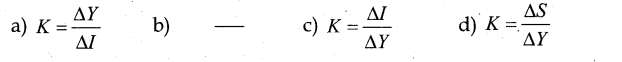Extra close brace or missing open brace

Question 8.
The formula for accelerator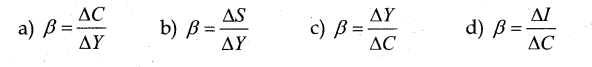β=ΔIΔC

Question 9.
The combined effect of the interaction of multiplier and accelerator is called ……………………
(a) Super accelerator
(b) Super multiplier
(c) Accelerator
(d) Multiplier
(b) Super multiplier

Question 10.
The systematic development of the simple accelerator model was made by …………..
a) J.M.Clark
b) Hawtrey
c) J.R.Hicks
d) J.M.Keynes
a) J.M.Clark

IX. Fill in the Blanks.

Question 1.

 Income Y Consumption (C) Savings S = Y – C 1. 120 120 0 2. 180 ? 10 3. 240 180 ?

C = 170, S = 60

Question 2.

 MPC MPS K 1) 0.00 1.00 1 2) 0.10 0.90 ?a 3) 0.50 ?b 2.00 4) 0.75 0.25 ?c 5) ?d 0.10 10.00 6) 1.00 0.00 ?e

a) 1.11 b) 0.5 c) 4 d) 0.90 e) 0

X. 2 Mark Questions

Question 1.
Write “Propensity to consume” Equations?

• The Average Propensity to Consume = cy
• The Marginal Propensity to Consume = ΔcΔy
• The Average Propensity to Save = xy
• The Marginal Propensity to Save = ΔsΔy

Question 2.
What is the investment function?
The investment function refers to investment – interest rate relationship. There is a functional and inverse relationship between rate of interest and investment.

Question 3.
Define “Laissez-Faire” – Capitalist Economy?
Existence of a Laissez-faire Capitalist Economy:
The law operates in a rich capitalist economy where there is no government intervention. People should be free to spend increased income. In the case of regulation of private enterprise and consumption expenditures by the State, the law breaks down.

Question 4.
What is the Marginal Efficiency of Capital?
MEC refers to the annual percentage yield earned by the last additional unit of capital.

Question 5.
Mention the factors that MEC depends on.

• The prospective yield from a capital asset.
• The supply price of a capital asset.

Question 6.
What is autonomous consumption?
Autonomous consumption is the minimum level of consumption or spending that must take place even if a consumer has no disposable income, such as spending for basic necessities.

Question 7.
Define “Autonomous consumption”?
Autonomous Consumption:
Autonomous consumption is the minimum level of consumption or spending that must take place even if a consumer has no disposable income, such as spending for basic necessities.

Question 8.
Name the factors affecting MEC.

• The cost of the capital asset.
• The expected rate of return during its lifetime.
• The market rate of interest.

Question 9.
State Keynes psychological law of consumption.
The law implies that there is a tendency on the part of the people to spend on consumption less than the full increment of income.

Question 10.
What are objective factors?
Objective factors are the external factors which are real and measurable.

Question 11.
What is the Leverage Effect?
The combined effect of the multiplier and the accelerator is called the leverage effect.

Question 12.
What is the Marginal Efficiency of Investment?
MEI is the expected rate of return on investment as additional units of investment are made under specified conditions and over a period of time.

Question 13.
Differentiate Positive and Negative Multiplier Effects.
Positive Multiplier: When an initial increase in an injection leads to a greater final increase in real GDP.
Negative Multiplier: When an initial increase is an injection leads to a greater final decrease in real GDP.

Question 14.
Mention the uses of Multiplier.

• Multiplier highlights the importance of investment in income and employment theory.
• The process throws light on the different stages of the trade cycle.
• It also helps is bringing equality between S and I.
• It helps in formulating Government Policies.
• It helps to reduce unemployment and achieve full employment.

XI. 5 Mark Questions

Question 1.
Name the determinants of investment functions?

• Rate of Interest
• Level of Uncertainty
• Political Environment
• Rate of growth of population
• The stock of Capital goods
• The necessity of new products
• Level of income of investors
• Inventions and innovations
• Consumer demand
• The policy of the state.

Question 2.
Explain the Keynes Psychological Law’ of consumption assumptions?
Keynes’s Law is based on the following assumptions:
1. Ceteris paribus (constant extraneous variables):
The other variables such as income distribution, tastes, habits, social customs, price movements, population growth, etc. do not change and consumption depends on income alone.

2. Existence of Normal Conditions:

• The law holds good under normal conditions.
• If, however, the economy is faced with abnormal and extraordinary circumstances like war, revolution, or hyperinflation, the law will not operate.
• People may spend the whole of increased income on consumption.

3. Existence of a Laissez-faire Capitalist Economy:

• The law operates in a rich capitalist economy where there is no government intervention.
• People should be free to spend increased income.
• In the case of regulation of private enterprise and consumption expenditures by the State, the law breaks down.

## How to Prepare using Samacheer Kalvi 12th Economics Chapter 4 Consumption and Investment Functions Notes PDF?

Students must prepare for the upcoming exams from Samacheer Kalvi 12th Economics Chapter 4 Consumption and Investment Functions Notes PDF by following certain essential steps which are provided below.

• Use Samacheer Kalvi 12th Economics Chapter 4 Consumption and Investment Functions notes by paying attention to facts and ideas.
• Pay attention to the important topics
• Refer TN Board books as well as the books recommended.
• Correctly follow the notes to reduce the number of questions being answered in the exam incorrectly
• Highlight and explain the concepts in details.

## Frequently Asked Questions on Samacheer Kalvi 12th Economics Chapter 4 Consumption and Investment Functions Notes

#### How to use Samacheer Kalvi 12th Economics Chapter 4 Consumption and Investment Functions Notes for preparation??

Read TN Board thoroughly, make separate notes for points you forget, formulae, reactions, diagrams. Highlight important points in the book itself and make use of the space provided in the margin to jot down other important points on the same topic from different sources.

#### How to make notes for Samacheer Kalvi 12th Economics Chapter 4 Consumption and Investment Functions exam?

Read from hand-made notes prepared after understanding concepts, refrain from replicating from the textbook. Use highlighters for important points. Revise from these notes regularly and formulate your own tricks, shortcuts and mnemonics, mappings etc.
Share: